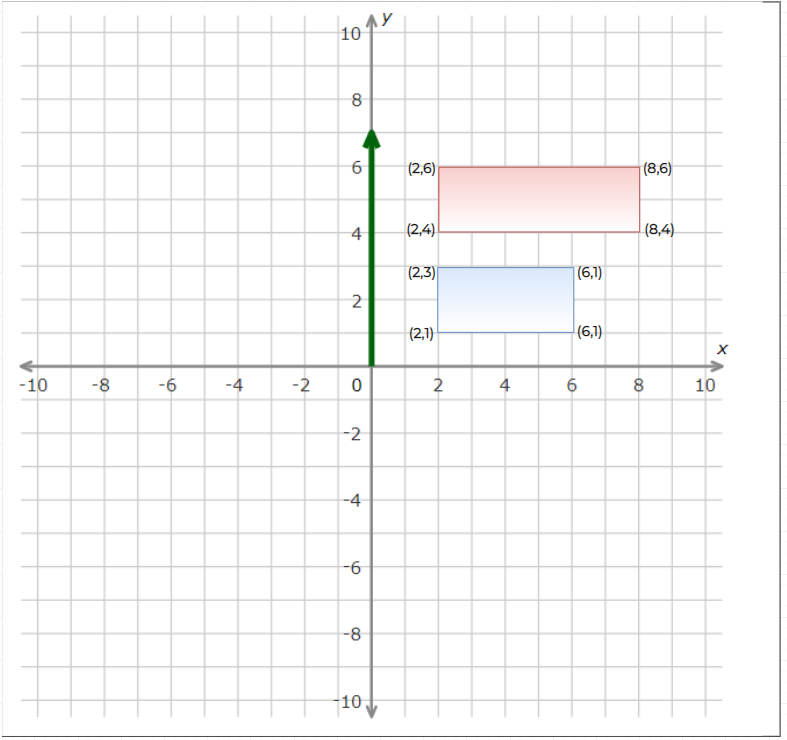## Find Minimum Area Rectangle

Given a set of points in the xy-plane, determine the minimum area of a rectangle formed from these points, with sides parallel to the x and y axes. If there isn't any rectangle, return 0.

Example 1:

```Input: [[1,1],[1,3],[3,1],[3,3],[2,2]]
Output: 4```

We need to count each rectangle by right-most edge.

1. Group the `y` points by `x` coordinates, so that we have columns of points. `<X,[List of Y]>`
2. Then, for every pair of points in a column (with coordinates `(x,y1)` and `(x,y2)`), check for the smallest rectangle with this pair of points as the rightmost edge. We can do this by keeping the memory of what pairs of points we've seen before.``````class Solution {
public int minAreaRect(int[][] points) {
if (points == null || points.length == 0) return 0;
int minArea = Integer.MAX_VALUE;
Map<Integer,List<Integer>> yListByX = new TreeMap<>();
for (int[] point: points) {
int x = point, y = point;
List<Integer> yList = yListByX.get(x);
if (yList == null) {
yList = new ArrayList<>();
yListByX.put(x, yList);
}
}
Map<String,Integer> seenX = new HashMap<>();
for (int x: yListByX.keySet()) {
List<Integer> yList = yListByX.get(x);
Collections.sort(yList);
for (int i = 0; i < yList.size(); i++) {
int y1 = yList.get(i);
for (int j = (i + 1); j < yList.size(); j++) {
int y2 = yList.get(j);
String key = y1 + "_" + y2;
Integer prevX = seenX.get(key);
if (prevX != null) {
minArea = Math.min(minArea, (x - prevX) * (y2 - y1));
}
seenX.put(key, x);
}
}
}
return minArea < Integer.MAX_VALUE ? minArea : 0;
}
}``````

Complexity Analysis

• Time Complexity: `O(N^2)`, where `N` is the length of `points`.

• Space Complexity: `O(N)`.

Arrays Algorithm